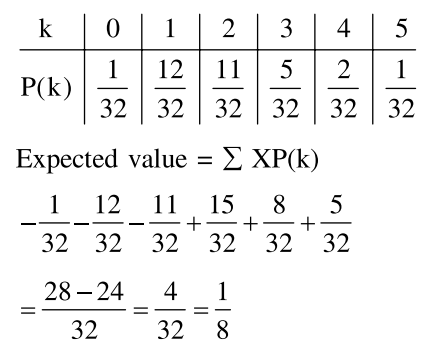# An unbiased coin is tossed 5 times.`
Question:

An unbiased coin is tossed 5 times. Suppose that a variable $\mathrm{X}$ is assigned the value $k$ when $k$ consecutive heads are obtained for $\mathrm{k}=3,4,5$ otherwise $X$ takes the value $-1$. Then the expected value of $X$, is :

1. $\frac{3}{16}$

2. $-\frac{3}{16}$

3. $\frac{1}{8}$

4. $-\frac{1}{8}$

Correct Option: , 3

Solution: